top of page

### P5JS projects

Duration six weeks

##### Basic function of P5JS, Sound and Image

let pos;
let vel;

let img;
let mySound;

###### Import sound and image

soundFormats('mp3','ogg')
}
function setup() {
let cnv = createCanvas(800,600);

cnv.mousePressed(canvasPressed);
background(200)
text ('tap to start/stop, 20, 40');

###### Setting speed and place

function canvasPressed(){
mySound. play();
}

colorMode(HSB, width,100,100)

//posX = width/2;
//posY = height/2;

pos = createVector(width/2, height/2);
vel = createVector(2, -1.7);
}

###### Draw the balls and move balls

function draw() {
background(width*2, 70, 80);

for(let i = 0; i < width; i++){
stroke(i,80,80)
line(i, 0, i, height);
}

image(img,0,0)

noStroke()
fill(pos.x,100, 100)

vel.x = vel.x * -1;
}
vel.y = vel.y * -1;
}
}# Rotate and Random

This week, working on the rotation of the graph, made it with random and noise color using code. Also the rotation of the graphs. Creates some interesting animation based on the basic logic of P5js.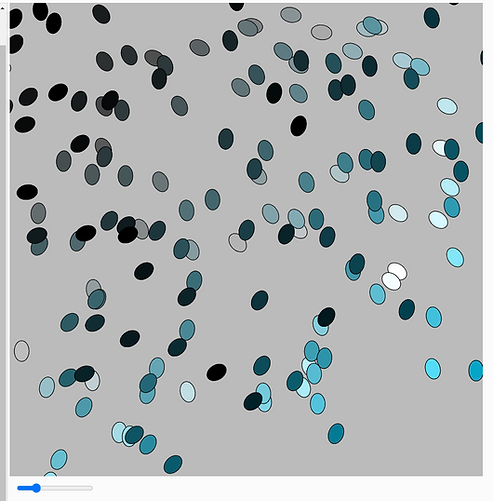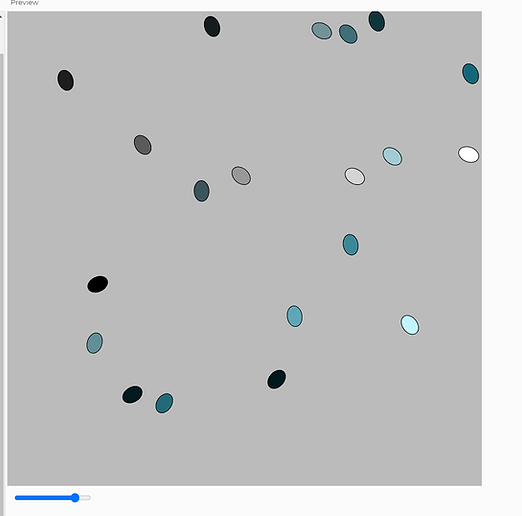# Function Draw Graph and fill color

Function draw() {
coeff = coeffControl.value()
background(220);

translate(width/2, height/2);

for(let i = 0; i < width/coeff; i++){
for(let j = 0; j <height/coeff; j++){
let sat = map(i, 0, (width/coeff) - 1, 0, 250);
let bri = map(j, 0, (height/coeff) - 1, 0, 300);

let coeff = 30
let randCoeff = 0.1;
let diam;
let ang = 0

let coeffControl;

function setup() {
createCanvas(800,800);

colorMode(HSB, 330, 250, 300)

coeffControl = createSlider(10,100, coeff, 5);
coeffControl.position(10,height+10);
}

By setup, the ColorMode in HSB can create a different feeling of color than RGB, the Saturation, and Brightness to make the graphs in different colors but in the same solar scheme.

Using rotate function in P5JS to make the graphs rotate in the canvas,

In the beginning, I make the rotate angle as + 0.01, and the graph is moving super fast.

But while the workshop, changing to a much smaller number of rotate angle as +0.0000001, finally can see how the graphs moving.

let nPx = map(i, 0, (width/coeff) - 1, 0, 1);
let nPy = j/(height/coeff)-1;

let x = i*coeff+coeff/2 + noise(nPx, nPy);

let y = j*coeff+coeff/2 + noise(nPx, nPy);

rotate(ang)
ang += 0.0000001;
fill(90, sat, bri);
rect (x, y, 25 ,35);

##### Class Function

Using the class function to create different classes.

The main tab can only use the class name to control the things inside the class.

Like draw a new circle or square, control their moves and changes.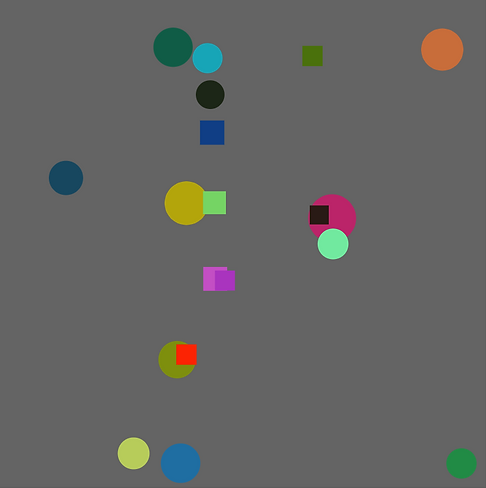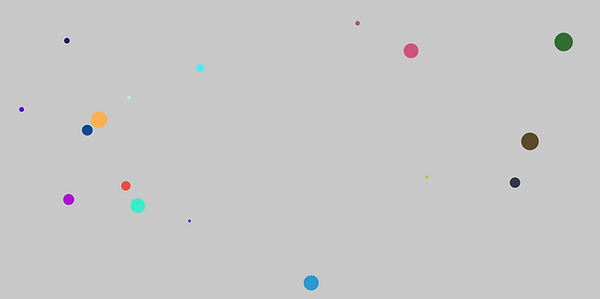Wish to create a Fish eat Fish game based on different shapes, and Circle means big fish, square means small fish.

The original idea is to circle able to eat square and grow bigger than before.

But the function  DISTANCE is not finished, because of the problem of Define the distance between circle and square.

Also, another problem is how to make the square go if the circle ate the square.

these are two main problems in this project.

let mySphere = [];
let mySquare = [];
let myTriangle = [];
let tol = 10

function setup() {
createCanvas(windowWidth, windowHeight);
mySphere.push(new Ball(width/2, height/2))
}

function draw(){
background(200);

mySphere.forEach((cir) =>{
cir.move();
cir.display();
})

mySquare.forEach((sqr) =>{
sqr.move();
sqr.display();
})

myTriangle.forEach((tri) =>{
tri.move();
tri.display();
})
}
function mouseReleased(){
mySphere.push(new Ball(random(width),random(height)));
}
function keyPressed(q){
mySquare.push(new Square(random(width),random(height)))

//function keyPressed(){
//    myTriangle.push(new Triangle(random(width),random(height)))

}

##### Main tab
###### Control the movement and generate new circles and squares.

class Square {

constructor (_x,_y){
this.x = random(width);
this.y = random(height)
this. pos = createVector(_x, _y);
this. ang = 0
this. vel = createVector(random(-5,5), random(-5,5));

this. rect = color(random(255), random(255), random(255));
}

display(){
noStroke();
fill(this.rect);
rect(this.pos.x, this.pos.y, random(30,40));
}

move(){
//translate(width/2,height/2);
//    rotate(this.ang);
//this.ang += 0.01;

if(this.pos.x+this.pos.y >= width || this.pos.x-10<= 0){
this.vel.x = this.vel.x * -1;
}
if(this.pos.y+this.pos.x >= height || this.pos.y-10 <= 0){
this.vel.y = this.vel.y * -1;

}
}
}

class Ball {

constructor (_x,_y){
this. pos = createVector(_x, _y);

this. vel = createVector(random(-4,4), random(-4,4));

this. clr = color(random(255), random(255), random(255));
}

display(){
noStroke();
fill(this.clr);
}

move(){

this.vel.x = this.vel.x * -1;
}
this.vel.y = this.vel.y * -1;
}
}
}

##### Teachable Machine

Using the teachable machine to create our own database, and able to analyze different modes with different definitions.

Wish to create the paper, scissors, rock game by using the database I created.

The problem is teachable machines have problems with function classifyVideo.

when I create the game, it is not able to connect with my database,

How to make a system randomly pick paper, scissors, rock is also a hard problem.

The moves are able to work, but the game cannot define win or lose.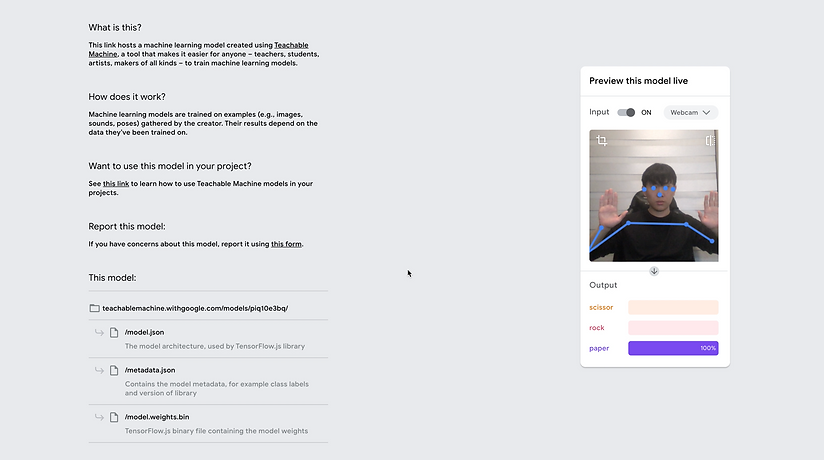###### import the teachable machine database to classify Video, and set variables for analysis.

let video;
let poseNet;
let poses = [];
let classifier;

function setup() {
createCanvas(640, 480);
video = createCapture(VIDEO);
video.size(width, height);
flippedVideo = ml5.flipImage(video);
// Start classifying
//classifyVideo();
classifier = ml5.neuralNetwork( + model.json );

// Create a new poseNet method with a single detection
// This sets up an event that fills the global variable "poses"
// with an array every time new poses are detected
poseNet.on('pose', function(results) {
poses = results;
});
;
// Hide the video element, and just show the canvas
video.hide();
}

// let options = {
// inputs: 34,
// outputs: 3,

//debug: ture}

const options = {
}
const nn = ml5.neuralNetwork(options);

const modelDetails = {
model: 'model.json',
weights: 'weights.bin'
}

// continue on your neural network journey
// use nn.classify() for classifications or nn.predict() for regressions
}

}
function mousePressed(){
console.log(JSON.stringify(poses))
}

function draw() {
image(video, 0, 0, width, height);

// We can call both functions to draw all keypoints and the skeletons
drawKeypoints();
drawSkeleton();
}

// A function to draw ellipses over the detected keypoints
function drawKeypoints()  {
// Loop through all the poses detected
for (let i = 0; i < poses.length; i++) {
// For each pose detected, loop through all the keypoints
let pose = poses[i].pose;
for (let j = 0; j < pose.keypoints.length; j++) {
// A keypoint is an object describing a body part (like rightArm or leftShoulder)
let keypoint = pose.keypoints[j];
// Only draw an ellipse is the pose probability is bigger than 0.2
if (keypoint.score > 0.2) {
fill(255, 0, 0);
noStroke();
ellipse(keypoint.position.x, keypoint.position.y, 10, 10);
}
}
}
}

###### Draw the skeleton of the pose, and make the skeleton able to define the pose.

function drawSkeleton() {
// Loop through all the skeletons detected
for (let i = 0; i < poses.length; i++) {
let skeleton = poses[i].skeleton;
// For every skeleton, loop through all body connections
for (let j = 0; j < skeleton.length; j++) {
let partA = skeleton[j];
let partB = skeleton[j];
stroke(255, 0, 0);
line(partA.position.x, partA.position.y, partB.position.x, partB.position.y);
}
}
}
function draw() {
background(0);
// Draw the video
image(flippedVideo, 0, 0);

if (poses.length > 0) {
let pose = poses.pose;

fill(213, 0, 143);
let rock = pose['rock'];

fill(255, 215, 0);
let paper = pose['paper'];

fill(255, 215, 0);
let scissor = pose['scissor'];

}
// }

function classifyVideo() {
flippedVideo = ml5.flipImage(video)
classifier.classify(flippedVideo, gotResult);
flippedVideo.remove();
}
function gotResult(error, results) {
// If there is an error
if (error) {
console.error(error);
return;
}
// The results are in an array ordered by confidence.
// console.log(results);
label = results.label;
// Classifiy again!
classifyVideo();
}
}

bottom of page